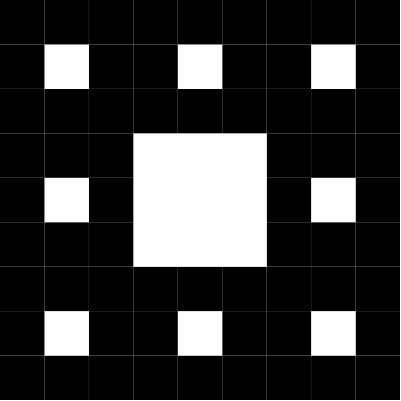This fractal is based on squares pattern below:solution

    ; Function sierpinskiGasket: size:Number, limit:Number -> Image
(cond
((<= size limit) (square size "solid" "black"))
(else (above
(beside
(beside
(square (/ size 3) "solid" "white")
(beside
(sierpinskiGasket 400 12)

Problem 2: Population estimate

A city's population is $$p$$ and increasing at a rate $$r$$ every year. For example Antalya's population was 1.800.000 in 2008 and increased at a rate of 0.12 (i.e. 12%) every year.

Write a function to find expected population in $$n\ge 0$$ years from now given current population $$p\ge 0$$ and increase rate $r <ge 0$.

Solution

    ;Function population p:Number, r:Number, n:Number
;Returns expected population in n years
;starting with p, and increase rate r
(define (population p r n)
(cond
((or (< n 0) (< p 0) (< r 0)) (error "invalid input"))
((= n 0) p)
(else (* (population p r (- n 1)) (+ 1 r)))))

(check-expect (population 1000 0.01 0) 1000)
(check-expect (population 1000 0.01 1) 1010)
(population 1000 0.01 10)